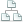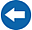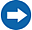Home >Tips >Excel 2016 from Scratch >Calculate Sums ASite map

# Excel 2016 from Scratch Exercise Calculate Sums A

## An Excel tutorial by Peter KalmstromIn this demo in the Excel 2016 from Scratch series, Peter Kalmstrom shows how to calculate sum in four different ways.

As an example, Peter uses sales of outdoor plants for different kinds of plants in different months.

### Exercise

If you want to try the exercise yourself, please download the Excel file Peter uses in the demo!

### Content

This is what Peter shows in the demo below:
• How to calculate the sum of all months of sales for a plant by using the AutoSum button.
• How to drag and drop cell content to another cell.
• How the mouse changes when it is moved around the selected area.
• How to calculate the sum of all months of sales for a plant by using a formula.
• How to calculate the sum of all months of sales for a plant by using the AutoSum button, but ignoring the default selection and instead selecting another range for the sum.
• How to fill formulas from one cell into the cells below with the help of little plus (+) symbol.
Peter uses Excel 2016 for his demo, but the Excel basics are the same for earlier versions of Excel.